# RFEM – Online Manual

Online manuals, introductory examples, tutorials, and other documentation.

# 4.1

General description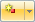The geometry of the model is defined by nodes. They are essential for creating lines, and thus members, surfaces, and solids. Every node is specified by its coordinates X, Y, Z. The coordinates usually refer to the origin of the global coordinate system but it is also possible to define them in relation to another node.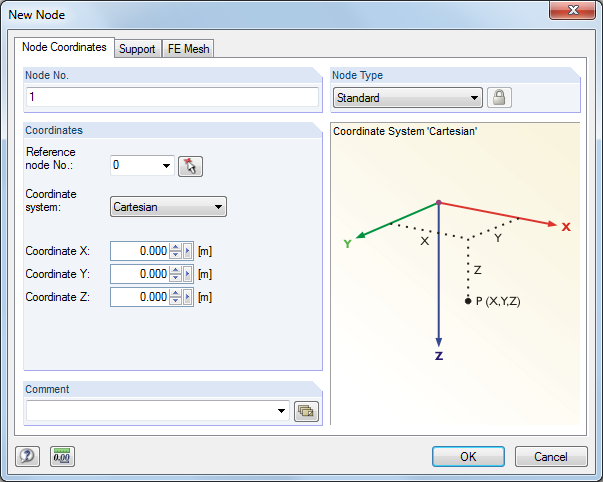Figure 4.11 New Node dialog box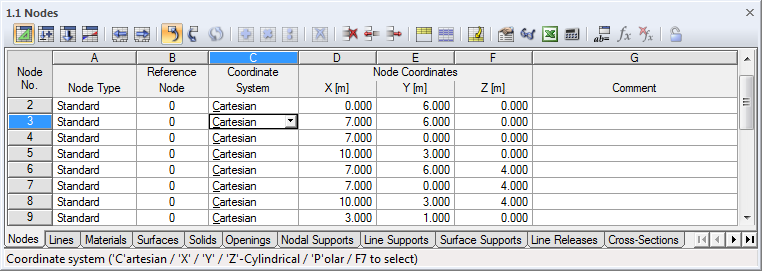Figure 4.12 Table 1.1 Nodes

The node number is automatically assigned in the New Node dialog box and can also be modified in it. The sequence of the node numbering is not important: Gaps in the numbering are permitted.

To adjust the order of node numbers retroactively, select Tools → Renumber on the menu (see Chapter 11.4.18).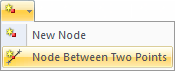The list button contains a special function. It allows you to create a node on the connection line of two existing nodes (see Chapter 11.4.12).

Node Type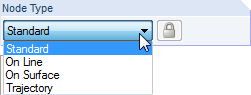Standard

This type of node is used most frequently. Standard nodes can be placed graphically in the work plane or anywhere in the workspace via coordinate specification. When you graphically enter lines or rotated surfaces, standard nodes are created.

Standard nodes are displayed in red in the graphic.

On Line

With this type of node, a line is not divided into two lines and remains complete instead. The nodal parameter δ describes the relative distance to the start node of the line.

By creating nodes on lines, it is possible to apply nodal loads anywhere on the line or to force an FE node.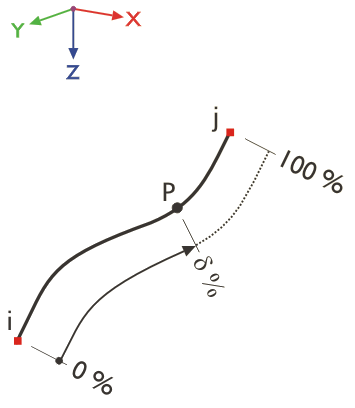Figure 4.13 Node on line

Nodes on lines are displayed in light blue by default.

By using the Tools → Generate Nodes on Intersection Point of Lines menu option, you can create nodes on lines that intersect.

On Surface

For quadrangle surfaces, it is difficult to determine the coordinates of nodes on the curved surface. With the Node on Surface node type, you can place a node directly on a quadrangle surface displayed in the graphic. The nodal parameters δ1 and δ2 refer to the four corner nodes of the surface.

By creating nodes on surfaces, it is possible to apply nodal loads anywhere on the curved surface or to force an FE node.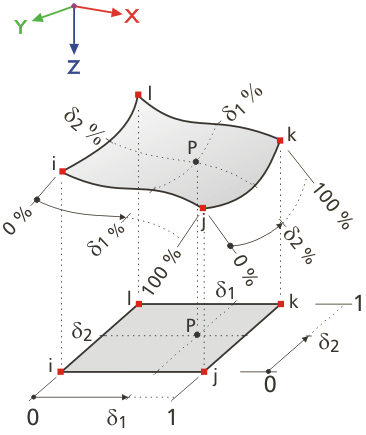Figure 4.14 Node on surface

Coordinates in the table are stored in the Cartesian coordinate system. Nodes on surfaces are graphically displayed in light blue by default.

If you work with plane surfaces, use standard nodes.

Trajectory

This type of node is created when you define a spiral trajectory curve (see Chapter 4.2). The nodal parameter δ describes the relative distance from the start node of the line.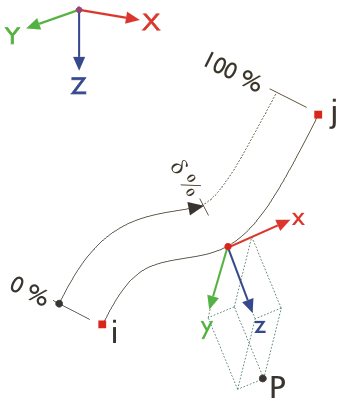Figure 4.15 Trajectory

Trajectory nodes are displayed in dark green by default.

Reference node

In general, the coordinates of a node are related to the origin 0 of the global coordinate system. You do not need to define the node (0/0/0) because RFEM automatically recognizes the origin.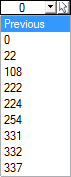Any node may serve as a reference node; even a node with a higher number is allowed to be used as a reference node. Referring to another node may be useful, for example, to define a new node at a certain distance to a known position. The table list with its Previous node option is especially useful in this case.

In the New Node dialog box, you can enter the reference node directly, select it from the list, or define it graphically using the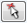button.

Coordinate system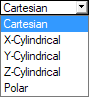The coordinates of a node always refer to a coordinate system that describes the position of the node in the workspace. Depending on the model geometry, you can select between different coordinate systems. All coordinate systems are oriented clockwise.

Cartesian

The global axes X, Y, and Z describe a translational expansion (linear). All directions of coordinates are on par with each other.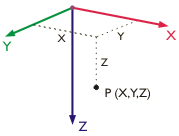Figure 4.16 Cartesian coordinate system

In most cases, nodes can be defined in the Cartesian coordinate system.

X-Cylindrical

The X-axis describes a translational expansion. The radius R defines the distance of the node to the X-axis. The angle θ defines the rotation of the coordinates about the X-axis.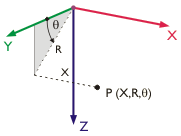Figure 4.17 X-cylindrical coordinate system

It is applied, for example, for tubular models whose central axis is the X-axis.

Y-Cylindrical

This coordinate system is similar to the X-cylindrical system, but in this case the longitudinal axis is represented by the Y-axis.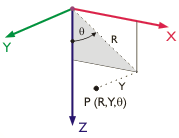Figure 4.18 Y-cylindrical coordinate system
Z-Cylindrical

The coordinate system is similar to the X-cylindrical system, but in this case the longitudinal axis is represented by the Z-axis.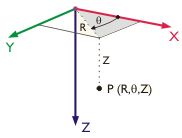Figure 4.19 Z-cylindrical coordinate system
Polar

In the polar coordinate system, the node position is described by a radius that specifies the distance to the point of origin and the angles θ and φ.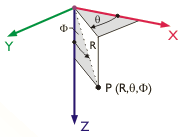Figure 4.20 Polar coordinate system

If possible, organize the model input with regard to the global coordinate system in such a way that the XYZ-axes of the coordinate system are in line with the principal directions of the modeled framework. This allows for an easier definition of coordinates, conditions, and loads.To define nodes directly in the workspace, open the floating New Node dialog box for graphical input. Usually, nodes snap to grid points that are aligned with the active user-defined or global coordinate system (CS).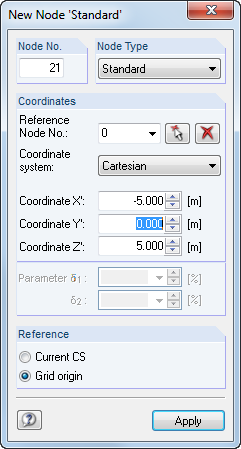Figure 4.21 Floating New Node dialog box

For more information about user-defined coordinate systems, see Chapter 11.3.4.

If the coordinate system is changed in the table, the node coordinates can be automatically converted to the new system. The following query is displayed.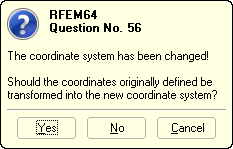Figure 4.22 RFEM query

You can analogously convert node coordinates with the Previous reference node in relation to the origin.

Node coordinates

Node coordinates are defined in the previously set coordinate system. When you model a 3D structure, the node is clearly defined by the coordinates X, Y, and Z, or the radius and angles. Coordinate parameters and table column titles change depending on the coordinate system.

When the model type is restricted to a 2D plate or wall, it is not possible to access all three text boxes or columns.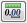To adjust Lengths and Angles, select Edit → Units and Decimal Places on the menu or use the corresponding button in the dialog box.

With the following procedure, you can check if all nodes of a surface lie in one plane: Select the relevant nodes and double-click one of them to open the Edit Node dialog box. Coordinate data is filled only in the text boxes whose values are conform for all selected nodes. If this is not the case, you can now assign a uniform plane coordinate to the selected nodes.

It is also possible to import node coordinates from Excel (see Chapter 11.5.6) or determine them with the formula editor of RFEM (see Chapter 11.6). In addition, there are several model generators that facilitate the input (see Chapter 11.7.2).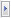To enter accurate, unrounded coordinates, select Full Precision in the New Node dialog box.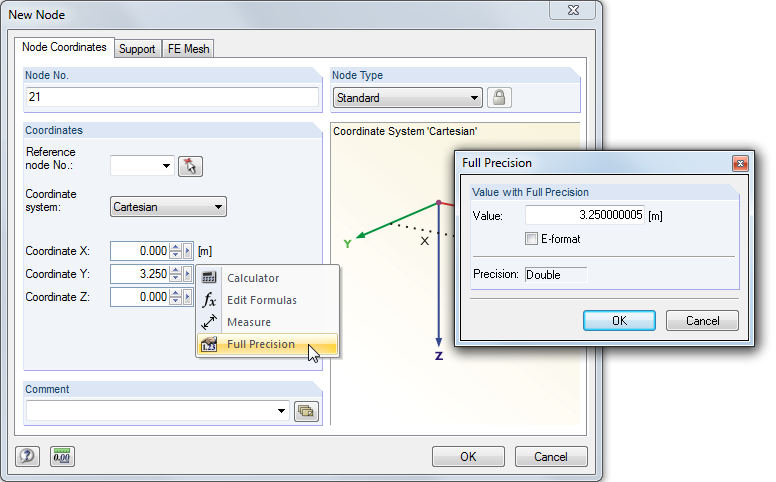Figure 4.23 Shortcut menu in the New Node dialog box and Full Precision dialog box
Comment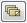This field allows you to enter user-defined notes. Use the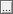button or [Apply Comment] to import saved comments (see Chapter 11.1.4).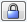A Generated comment is displayed for nodes that are generated by RFEM when creating an intersection or a rotated surface. Click the button shown on the left, available in the dialog box and table, to "unlock" generated nodes and make them accessible for modifications.